# Ray And Wave Optics Practice Level - 1

## 30 Questions MCQ Test Exclusive Video Lectures of Class 12 Physics by Experts | Ray And Wave Optics Practice Level - 1

Description
This mock test of Ray And Wave Optics Practice Level - 1 for Class 12 helps you for every Class 12 entrance exam. This contains 30 Multiple Choice Questions for Class 12 Ray And Wave Optics Practice Level - 1 (mcq) to study with solutions a complete question bank. The solved questions answers in this Ray And Wave Optics Practice Level - 1 quiz give you a good mix of easy questions and tough questions. Class 12 students definitely take this Ray And Wave Optics Practice Level - 1 exercise for a better result in the exam. You can find other Ray And Wave Optics Practice Level - 1 extra questions, long questions & short questions for Class 12 on EduRev as well by searching above.
QUESTION: 1

Solution:
QUESTION: 2

Solution:
QUESTION: 3

### You are given two identical plano-convex lenses. Whenyou place an object 20 cm to the left of a single planoconvexlens, the image appears 40 cm to the right ofthe lens. You then arrange the two plano-convex lensesback to back to form a double convex lens. If the objectis 20 cm to the left of this new lens, what is theapproximate location of the image?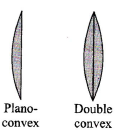Solution:
QUESTION: 4

A man is walking under an inclined mirror at a constantvelocity Vms-1 along the X-axis. If the mirror is inclinedat an angle θwith the horizontal, then what is thevelocity of the image?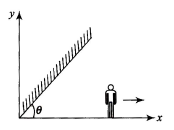Solution:
QUESTION: 5

A ray of light passes from glass, having a refractive index of  1.6, to air. The angle of incidence for which the angle of refraction is twice the angle of incidence is

Solution:
QUESTION: 6

Consider an equiconvex lens of radius of curvature Rand focal length f. If f > R, the refractive index μ of thematerial of the lens

Solution:
QUESTION: 7

Consider an equiconvex lens of radius of curvature Rand focal length f. If f > R, the refractive index μ of thematerial of the lens

Solution:
QUESTION: 8

A fish is vertically below a flying bird moving verticallydown toward water surface. The bird will appear to thefish to be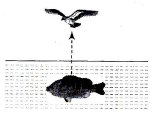Solution:
QUESTION: 9

Wh at is the angle of incidence for an equilateral prismof refractive index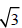so that the ray is parallel to thebase inside the prism?

Solution:
QUESTION: 10

A cube of side 2 m is placed in front of a concave mirror of focal length 1 m with its face A at a distance of 3 m and face B at a distance of 5 m form the mirror.The distance between the images of faces A and Band heights of images of A and B are, respectively,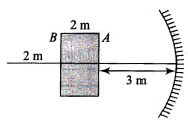Solution: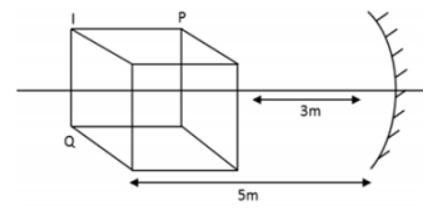For point ‘P ’ 1/Vp - 1/3 = -1/1 ⇒ 1/Vp = -1 + 1/3

Vp =  - 3/2 m

For point ‘Q ’  1/Vp - 1/5 = -1/1 ⇒1/Vq = -1 + 1/5

Vq = -5/4

So Vp - ­ VQ = 0.25m

Height of image of ‘P ’ ⇒ m = -v/u = h1/h0

= - (+3/2/+ 3) = h1/2m ⇒ h1 = - 1/2m

Height of image ‘Q’  ⇒ m = -(-5/4/5)

= h1/2m

h1 = 0.25m

So height of image of Q pace = 0.5m

Height of image of P pace = 1.0m

QUESTION: 11

A plano-convex lens when silvered on the plane sidebehaves like a concave mirror of focal length 60 cm.However, when silvered on the convex side, it behaveslike a concave mirror of focal length 20 cm. Then, therefractive index of the lens is

Solution:
QUESTION: 12

Two thin lenses are placed 5 cm apart along the sameaxis and illuminated with a beam of light parallel tothat axis. The first lens in the path of the beam is aconverging lens of focal length 10 cm whereas thesecond is a diverging lens of focal length 5 cm. If thesecond lens is now moved toward the first, theemergent light

Solution:
QUESTION: 13

An object is placed 30 cm to the left of a diverging lenswhose focal length is of magnitude 20 cm. Which oneof the following correctly states the nature and positionof the virtual image formed ?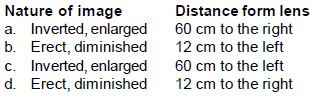Solution:
QUESTION: 14

A lens forms a real image of an object. The distancefrom the object to the lens is x cm and that from thelens to the image is y cm. The graph (see Fig.) showsthe variation of y with x.It can be deduced that the lens is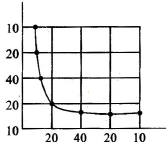Solution:
QUESTION: 15

A point source S is placed at the bottom of differentlayers as shown in Fig. The refractive index of thebottom most layer is μ0. The refractive index of anyother upper layer is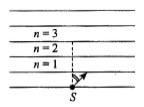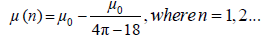ray of light starts from the source S as shown. Totalinternal reflection takes place at the upper surface of alayer having n equal to

Solution:
QUESTION: 16

A concave mirror has a focal length of 20 cm. Thedistance between the two positions of the object forwhich the image size is double of the object size is

Solution:
QUESTION: 17

A concave mirror has a focal length of 20 cm. Thedistance between the two positions of the object forwhich the image size is double of the object size is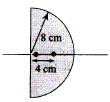Solution:
QUESTION: 18

A ray of light falls on a transparent sphere with centreat C as shown in Fig.The ray emerges from the sphere parallel to line AB.The refractive index of the sphere is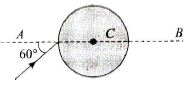Solution:
QUESTION: 19

A circular beam of light of diameter d= 2 cm falls on aplane surface of glass. The angle of incidence is 60°and refractive index of glass is μ = 3/2. The diameter ofthe refracted beam is

Solution:
QUESTION: 20

A ray of light is incident on a glass sphere of refractiveindex 3/2. What should be the angle of incidence sothat the ray which enters the sphere does not comeout of the sphere?

Solution:
QUESTION: 21

An object is kept at a distance of 16 cm from a thinlens and the image formed is real. If the object is keptat a distance of 6 cm from the same lens, the imageformed is virtual. If the sizes, of the images formed areequal, the focal length of the lens will be

Solution:
QUESTION: 22

A concave lens forms the image of an object such thatthe distance between the object and image is 10 cmand the magnification produced is 1/4. The focal lengthof the lens will be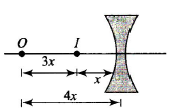Solution:
QUESTION: 23

A diver in a swimming pool wants to signal his distressto a person standing on the edge to the pool by flashinghis water proof flash light

Solution:
QUESTION: 24

A, B, and C are three optical media of respective criticalangles C1, C2, and C3. Total internal reflection of lightcan occur from A and B, also from B to C but not fromC to A. Then, the correct relation between the criticalangles is

Solution: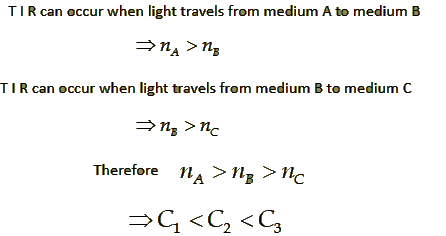QUESTION: 25

A point object O is placed on the principal axis of aconvex lens of focal length 20 cm at a distance of 40cm to the left of it. The diameter of the lens is 10 cm.If the eye is placed 60 cm to the right of the lens at adistance h below the principal axis, then the maximumvalue of h to see the image will be

Solution:
QUESTION: 26

Light of wavelength 500 nm traveling with a speed of 2.0 x 108 ms-1 in a certain medium enters anothermedium of refractive index 5/4 times that of the firstmedium. What are the wavelength and speed in thesecond medium?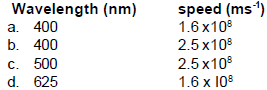Solution:
QUESTION: 27

A hollow double concave lens is made of very thin transparent material. It can be filled with air or either of two liquids L1 or L2 having refractive indices n1 and nrespectively (n2 > n1 > 1). The lens will diverge parallel beam of light if it is filled with

Solution:
QUESTION: 28

A convex lens of focal length 20 cm and a concavelens of focal length f are mounted coaxially 5 cm apart.Parallel beam of light incident on the convex lensemerges from the concave lens as a parallel beam.Then, f in cm is

Solution:
QUESTION: 29

An equiconvex lens is made from glass of refractive index 1.5. If the radius of each surface is changed from 5 cm to 6 cm, then the power

Solution:
QUESTION: 30

A concave lens made of water (μ = 1.33) is placed inside a slab (μ = 1.5) for an object placed between the focus and twice the focus. The image formed is

Solution: